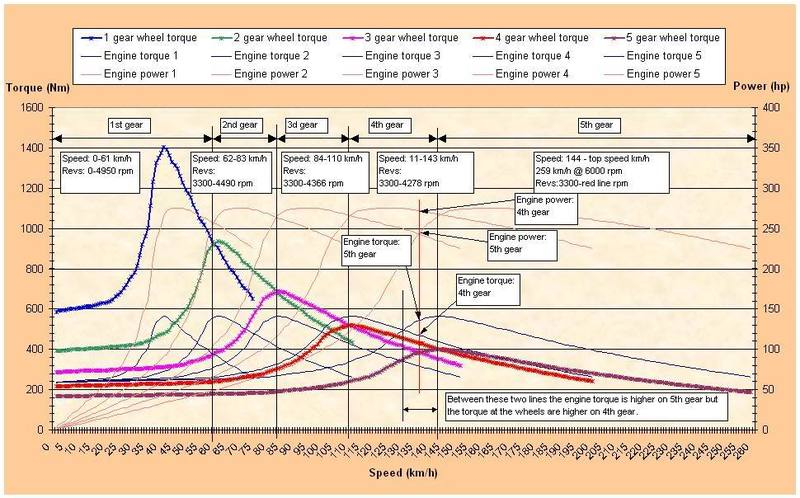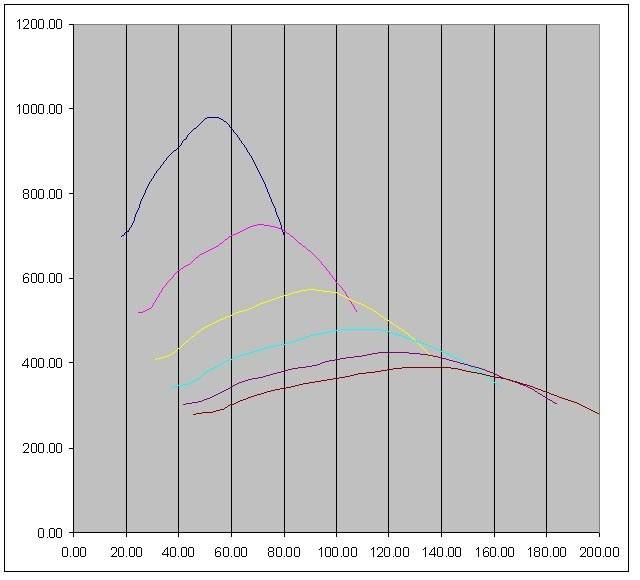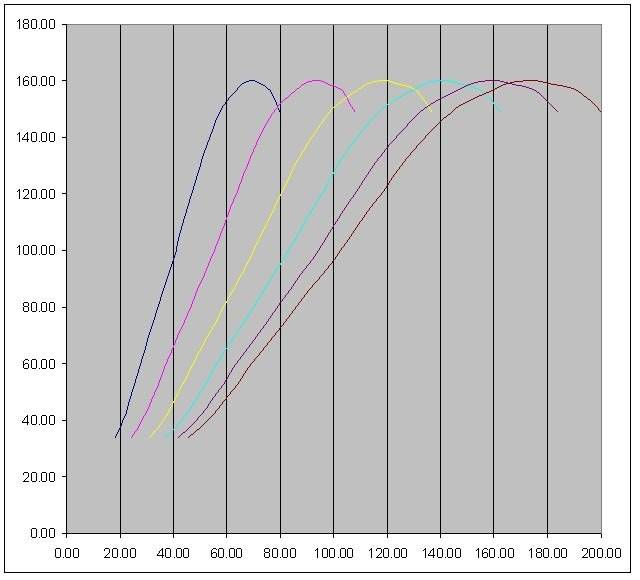# Calculating the rpms at which gear changes must occur: The Power Band

(To the moderator,please don't shift to the Homework thread list.This thread explores the conceptual part of gear changes)
A 2 liter engine has T(max)=185Nm at $\omega_e$=4500 rpm, has a manual gearbox with the following gear ratios:
n1=3.42,
n2=2.14,
n3=1.45,
n4=1.03,
n5=0.81,
nr=3.46,
nd=4.06; (final gearing ratio,has to be included in all calculations)
I find the radius of the car tires as 0.319m(as this is a Ford Mondeo which uses tires specified as 205/55/R16). I am asked to determine the gear speed equations for each gear:
$$\omega_e=\frac{n_in_d vx}{R_w}$$
$$T_e=\frac{R_wF_x}{\eta n_in_d}$$

I'm assuming $\eta$, the driveline efficiency is 1.
(My attempted solutions and understandings are attached as thumbnails)
Q.My question is on what rpm range do I base my power band(i.e the min and max rpms between which the gears change) $\omega_1$$\omega_2$?

I'm thinking of using the minimum rpm at which the driver must gear down as 4500rpm(i.e the rpm value at Torque(max)), the reasoning for this is at the end. But,I do not know the maximum rpm at which the driver must gear-up(which I presume is the rpm at max power ??). How do I find this out?

Normally, I can find the rpms at which the gear changes must occur from the rpm at which max.power $\omega_{Pmax}$ occurs. i.e I use this value of this rpm to then calculate the $\omega_1, \omega_2$ at which the gear changes must occur, by using the equation $\omega_{Pmax}=\frac{\omega_1+\omega_2}{2}$.

This excerpt was taken from a very good article I read and it related to my idea of using the minimum rpm at which gearing down must occur$\omega_1$ as the max torque rpm$\omega_{Pmax}$:
A vehicle engine operating at a rotational speed above its maximum-torque point is in a "stable" speed regime. If it slows down by a small amount (due to the vehicle encountering an incline, head-wind, etc.) engine torque will tend to increase and resist the slowing. Conversely, if it speeds up by a small amount, torque will tend to decrease and discourage a further increase in speed.

#### Attachments

Last edited:

jack action
Gold Member
To get a smooth transition, power must be the same before and after shifting.

If you are looking for maximum performance, the ω1 & ω2 should be before and after maximum power (like in your drawing) such that the average power will be at its highest. The gear ratios will determine the width of your power band width (ω2 - ω1)

In any other case, you will have to vary the throttle position to achieve the same (partial) power output before and after shifting.

For maximum fuel economy, it should be based similarly on the BSFC map.

Jack Action: So,for maximum fuel economy, one would design a gearbox with shift points centred at the max power.(?I'm confused because the BSFC map says the engine is most efficient around max torque point).

THEN taking the case for max.acceleration, would the shift points be centred around the max. torque point?(I'm not getting the same power value at either side of the shift points in these cases though).

Last edited:
jack action
Gold Member
Jack Action: So,for maximum fuel economy, one would design a gearbox with shift points centred at the max power.(?I'm confused because the BSFC map says the engine is most efficient around max torque point).

For maximum performance, your shift points are centered at the max power; For maximum fuel economy, your shift points are centered where you get best BSFC (usually centered at a point close to max torque).

THEN taking the case for max.acceleration, would the shift points be centred around the max. torque point?(I'm not getting the same power value at either side of the shift points in these cases though).

For maximum acceleration, the maximum wheel torque, at any given wheel RPM, will always be at max power; Except in first gear, where it will be at maximum torque (because there is no lower gear ratio available). Do the calculations, you'll see that you will always get less torque at the wheel when you're at maximum engine torque in a given gear ratio at a given wheel rpm than at maximum engine power in a lower gear ratio, given the same wheel rpm.

Did you mean:
Do the calculations, you'll see that you will always get less torque at the wheel when you're GEAR SHIFT POINTS ARE CENTRED at maximum engine torque in a given gear ratio at a given wheel rpm than at GEAR SHIFT POINTS BEING CENTRED at maximum engine power in a lower gear ratio, given the same wheel rpm.

I'm guessing the below image is for gear shifts around max power(Edit: I was right, as the website suggestshttp://www.motorsportforums.com/wrc/127068-wrc-group-n-torque.html, interestingly the lower shift point is at max.torque rpm for WRC cars).jack action
Gold Member
Did you mean:

I meant what I wrote. Proof:

Tn = torque at the wheel in nth gear at max engine torque;
Tn-1 = torque at the wheel in (n-1)th gear at max engine power;
nn = nth gear ratio;
nn-1 = (n-1)th gear ratio;
wp = engine rpm at max power;
wt = engine rpm at max torque;
Pp = engine max power;
Pt = engine power at max torque;

Tn = Pt / wp * nn
Tn-1 = Pp / wt * nn-1

For the car to be at the same velocity, i.e. the wheel rpm (ww) is the same, this condition must be true:

ww = wp / nn = wt / nn-1

So:

Tn = Pt / wt * nn-1 = Pt / wp * nn
Tn-1 = Pp / wt * nn-1 = Pp / wp * nn

Since Pp > Pt then Tn-1 > Tn, always.

Simply said:

Tw = Pw / ww

Since power at the wheel (Pw) is the same as the engine power, For a given wheel rpm (i.e. car velocity), the torque at the wheel (Tw) is proportional to the engine power, not the engine torque.

rcgldr
Homework Helper
I'm guessing the below image is for gear shifts around max power.
That chart shows gear shifts that should occur when the driven wheel torque curves for each gear cross (which is why those curves are colored). These are the shift points for maximum acceleration, so they are done to provide maximum driven wheel torque while accelerating. The chart assumes that shifts are nearly instant. If the shift takes some amount of time, you shift a bit later so that speed lost during a shift is compensated for.

The chart has a mistake in one of the labels: "engine power 5th gear" is really "engine power 3rd gear".

Last edited:
Straight off the bat, I am not an engineer of any description, but have had a very broad an involved interest in most things automotive for the past 25 years.
You guys all seem to be missing a critical point in all of you calculations. Wind resistance!

But before I go into that, because the gearbox, diff and wheels work as a gear reduction (multiplier of torque) the maximum amount of torque available at the wheels is DIRECTLY related to where the engine makes maximum torque, not power.
If the engine develops 200Nm of torque at 3000rpm, that means at 3000rpm there will be 200Nm multiplied by the reduction from the gearing, whether it be 3:1 or 5:1 ect ect.
For all intents and purposes you can ignore engine power.

To work out the best shift points, you need to have a torque curve of the engine, and overlay that with the torque at the wheels required to maintain a given speed. The power required to move through the air goes up at the square of the increase in speed. It may require in the ball park of 10kw to cruise at 100kmh, double that to 200kmh and you need 100kw. So the shift points can't be calculated from ratios and torque curve only, you must subtract the torque needed to maintain present speed from the the torque available at the wheels at a certain rpm. Given the coefficient of drag particular car, you should be able to calculate (within reason) the amount of drag (therefor torque), a particular car needs to travel at speed.
You will find doing a more elaborate calculation like this will have the engine needing to be reved more and more past the peak power to gain the best acceleration, all the way up until you start hitting the rev limiter in 4th and possibly 3rd to get the highest possible net torque at the wheels.

Damo

Damo,I think I can summarise your post with 1 equation:
$$F_x-F_R=m\frac{\mathrm{dv} }{\mathrm{d} t}$$

and the need for maximum acceleration can be quantified in terms of time needed by interchanging the above expression as:
$$t1=\int_{v1}^{v2}\frac{m}{F_x-F_r}dv$$

where, as Damo said, the traction force $F_x$ can be expressed as a function of velocity $F_x=f(velocity)$.

Thanks guys. Keep this thread open and I will come back with some data and calculations.

I am having a little trouble working out the numbers to go into that equation, could you plug in some figures to make it work?

http://www.autospeed.com/cms/A_111147/article.html

Damo

rcgldr
Homework Helper
For a given engine and transmission, and assuming the goal is maximum acceleration, you only need the driven wheel torque versus speed curve for each gear. The ideal shift points for maximum acceleration occur where these torque curves cross (at the highest speed cross over point if they cross more than once) (or shift at max rpm if the curves do not cross). Choosing these shift points will provide the driven wheels with the maximum possible torque at any speed.

The power band and other parameters of a vehicle are used when designing the vehicle components, such as choosing the set of gear ratios to use for a transmission, but the optimum acceleration shift points will continue to remain where the driven wheel torque versus speed curves in each gear cross.

jack action
Gold Member
Straight off the bat, I am not an engineer of any description, but have had a very broad an involved interest in most things automotive for the past 25 years.
You guys all seem to be missing a critical point in all of you calculations. Wind resistance!

It doesn't matter. Having more wind resistance will leave less room for acceleration, but it won't give you more wheel torque.

But before I go into that, because the gearbox, diff and wheels work as a gear reduction (multiplier of torque) the maximum amount of torque available at the wheels is DIRECTLY related to where the engine makes maximum torque, not power.
If the engine develops 200Nm of torque at 3000rpm, that means at 3000rpm there will be 200Nm multiplied by the reduction from the gearing, whether it be 3:1 or 5:1 ect ect.
For all intents and purposes you can ignore engine power.

The huge difference of having 200 N.m with a gear ratio of 3:1 vs a gear ratio of 5:1 is that, yes you will have a wheel torque of 600 N.m vs 1000 N.m but at a cost: In the 3:1 gear ratio the wheel will turn 1000 rpm and with 5:1 it will turn 600 rpm. That being said, if with the 3:1 gear ratio you're driving 50 km/h, with the 5:1 gear ratio, you're only at 30 km/h. The speed has to be the same to compare the maximum torque you can get from the engine, because the torque available at the wheel will always drop as the speed increase.

Let's see some numbers. The 2014 Corvette engine has the following peak values:

peak hp: 343 kW @ 6000 rpm (or 545 N.m @ 6000 rpm)
peak torque: 630 N.m @ 4600 rpm (or 303 kW @ 4600 rpm)

Let's say you have a 2:1 gear ratio. At peak torque you get 1260 N.m and your wheel rpm is 2300 rpm. At peak hp, you get only 1090 N.m, but you are at 3000 rpm.

Let's say you are now in a lower 2.608:1 gear ratio. At peak torque you get 1643 N.m and your wheel rpm is 1764 rpm. At peak hp, you get 1421 N.m, but you are at 2300 rpm.

Of course, in the lower gear ratio, peak torque still gives a higher wheel torque than peak hp, but they are still at a different wheel rpm. Now, notice that at 2300 rpm, you get more wheel torque in the lower gear ratio at peak hp than at peak torque in the higher gear ratio. That is why power is all that matter.

To get the most out of your engine - performance wise - you need to keep that engine close to peak hp as much as possible. And to get the smooth transition, the power before and after shifting gears has to stay the same, as in the graph in post #6.

rcgldr
Homework Helper
Here are two graphs for a 2001 Suzuki Hayabusa motorcycle. The first graph shows rear wheel torque versus speed in each gear. The second graph shows rear wheel power versus speed in each gear. The ideal shift points occur where the curves cross.You shift as soon as the wheel torque in the next gear is higher than in the current gear. This is the same thing as when the engine power is higher in the next gear than the current.